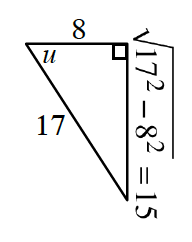### Home > PC3 > Chapter Ch8 > Lesson 8.3.3 > Problem8-122

8-122.

Angles $u$ and $v$ are in the same quadrant. If $\cos(u)=\frac{8}{17}$ and $\sin(v)=-\frac{9}{41}$, evaluate:

If cosine is positive and sine is negative, which quadrant are $u$ and $v$ in?

Draw a triangle diagram for each angle. One is done for you.1. $\tan\left(u + v\right)$

$\frac{\tan(u)+\tan(v)}{1-\tan(u)\tan(v)}$

$\frac{\left(\frac{-15}{8}\right)+\tan(v)}{1-\left(\frac{-15}{8}\right)\tan(v)}$

1. $\cos\left(v – u\right)$

$\cos(v)\cos(u)+\sin(v)\sin(u)$

$\cos(v)\left(\frac{8}{17}\right)+\sin(v)\left(\frac{-15}{17}\right)$